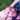# Block Objects in iOS

November 25, 2013

I need to put this here so I have a reference I can look up when I forget.

Block objects can either be inline or coded as independent blocks of code. Let’s start with the latter type. Suppose you have a method in Objective-C that accepts two integer values of type NSInteger and returns the difference of the two values, by subtracting one from the other, as an NSInteger:

``````- (NSInteger) subtract:(NSInteger)paramValue from:(NSInteger)paramFrom {
return paramFrom - paramValue;
}``````

pure C function that provides the same functionality to get one step closer to learning the syntax of block objects:

``````NSInteger subtract(NSInteger paramValue, NSInteger paramFrom){
return paramFrom - paramValue;
}``````

You can see that the C function is quite different in syntax from its Objective-C counterpart. Now let’s have a look at how we could code the same function as a block object:

``````NSInteger (^subtract)(NSInteger, NSInteger) = ^(NSInteger paramValue,
NSInteger paramFrom) {
return paramFrom - paramValue;
};``````

Suppose we have a function in C that takes a parameter of type NSUInteger (an unsigned integer) and returns it as a string of type NSString. Here is how we implement this in C:

``````NSString* intToString (NSUInteger paramInteger) {
return [NSString stringWithFormat:@"%lu",
(unsigned long)paramInteger];
}``````

The block object equivalent of this C function is:

``````NSString* (^intToString)(NSUInteger) = ^(NSUInteger paramInteger) {

NSString *result = [NSString stringWithFormat:@"%lu",(unsigned long)paramInteger];
return result;
};``````

The simplest form of an independent block object would be a block object that returns void and does not take in any parameters:

``````void (^simpleBlock)(void) = ^{
/* Implement the block object here */
};``````

Block objects can be invoked in the exact same way as C functions. If they have any parameters, you pass those as you would for a C function, and any return value can be retrieved exactly as you would retrieve a C function’s return value. Here is an example:”

``````NSString\* (^intToString)(NSUInteger) = ^(NSUInteger paramInteger) {
NSString *result = [NSString stringWithFormat:@"%lu",(unsigned long)paramInteger];
return result;
};

- (void) callIntToString {
NSString *string = intToString(10);
NSLog(@"string = %@", string);
}``````

The `callIntToString` Objective-C method is calling the `intToString` block object by passing the value 10 as the only parameter to this block object and placing the return value of this block object in the string local variable.”Written by Ilteris Kaplan who lives and works in New York. Twitter